# Partial differentiation

## Summary and examples

So far we have discussed how a function of one independent variable can be differentiated. But also functions with two or more independent variables can be differentiated.

Suppose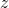is a function of two independent variablesen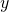, so we write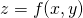.

The graph of this function is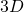.
The derivative toin a point of the graph of the function is the slope of the tangent line in that point in the-direction (i.e.is kept constant). For this so-called partial derivative various notations are used: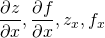.

The derivative toin a point of the graph of the function is the slope of the tangent line in that point in the-direction (i.e.is kept constant). For this so-called partial derivative various notations are used: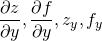.

The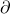instead of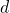indicates that we have to do with a partial derivative, either toor to.

#### How do we calculate a partial derivative?

In order to calculate the partial derivative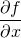, we consideras an independent variable andas a constant, so pretend thatis a constant. This is not really difficult, but you must be careful because a mistake is easily made.

##### Example 1

The function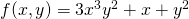has two independent variables,en.

If we consideras a constant, then the partial derivative tois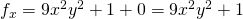.

##### Example 2

In this example not only the first, but also the second derivative is calculated.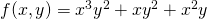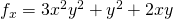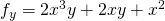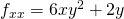(second partial derivative to)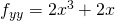(second partial derivative to)

There are also so-called mixed partial derivatives, first tonext to: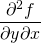; or first toand next to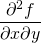.

For usual functions the mixed partial derivatives are equal: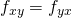. Just check this in this example.

0